Accounting Equation

• CompasX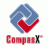CompasX

Guest
ACCOUNTING EQUATION is a mathematical expression used to describe the relationship between the assets, liabilities and owner's equity of the business model. The basic accounting equation states that assets equal liabilities and owner's equity, but can be modified by operations applied to both sides of the equation, e.g., assets minus liabilities equal owner's equity.

N

newworld

Guest
ACCOUNTING EQUATION: PHƯƠNG TRÌNH KẾ TOÁN

PHƯƠNG TRÌNH KẾ TOÁN là một biểu thức toán học dùng để mô tả mối quan hệ giữa tài có, công nợ và vốn góp của một mô hình doanh nghiệp. Phương trình kế toán cơ bản được biểu thị như sau: tài sản có = công nợ + vốn góp. Phương trình này có thể thay đổi khi thực hiện các phép toán ở hai vế của phương trình, ví dụ: vốn góp = tài sản có - công nợ.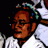Vualua

Geosynthetics
ACCOUNTING EQUATION is a mathematical expression used to describe the relationship between the assets, liabilities and owner's equity of the business model. The basic accounting equation states that assets equal liabilities and owner's equity, but can be modified by operations applied to both sides of the equation, e.g., assets minus liabilities equal owner's equity.

Tiếng Việt :
Phương trình kế toán là một biểu thức toán học được sử dụng để mô tả mối quan hệ giữa tài sản, các khoản nợ phải trả và vốn chủ sở hữu của một tổ chức kinh doanh. Phương trình kế toán căn bản cho thấy Tài sản luôn bằng các khoản nợ phải trả và vốn chủ sở hữu, nhưng có thể thay đổi hai vế của phương trình trong mỗi tình huống cụ thể .. VD : Tài sản trừ đi các khoản nợ phải trả luôn bằng vốn chủ sở hữu

Sửa lần cuối:
N

ngochb

Sơ cấp
Accounting equation: The formula used to prepare a balance sheet: assets=liability+equity.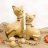Trả lời
7
Lượt xem
1K
Tiếng Anh Kế toán
THUY NGUYEN ACCA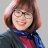W
Trả lời
0
Lượt xem
5K
W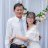Trả lời
0
Lượt xem
851J
Trả lời
0
Lượt xem
1K
J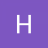Trả lời
1
Lượt xem
4KChia sẻ